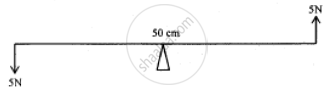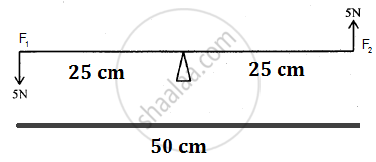Share

# Two Forces Each of 5n Act Vertically Upwards and Downwards Respectively on the Two Ends of a Uniform Metre Rule Which is Placed at Its Mid-point as Shown in the Diagram. Determine the Magnitude of the Resultant Moment of These Forces About the Midpoint. - ICSE Class 10 - Physics

ConceptMachines as Force Multipliers

#### Question

Two forces each of 5N act vertically upwards and downwards respectively on the two ends of a uniform metre rule which is placed at its mid-point as shown in the diagram. Determine the magnitude of the resultant moment of these forces about the midpoint.#### SolutionMoment of force F1 = 5 ⨯ 25 = 125
Moment of force F2 = 5 ⨯ 25 = 125
Since both the forces are acting in anticlockwise direction, the resultant moment of force is the sum of the two moments.
∴ Resultant moment of force = 125 + 125 = 250

Is there an error in this question or solution?

#### APPEARS IN

Solution Two Forces Each of 5n Act Vertically Upwards and Downwards Respectively on the Two Ends of a Uniform Metre Rule Which is Placed at Its Mid-point as Shown in the Diagram. Determine the Magnitude of the Resultant Moment of These Forces About the Midpoint. Concept: Machines as Force Multipliers.
S